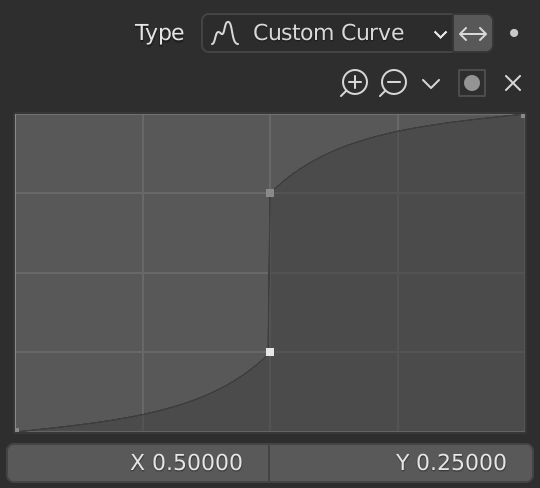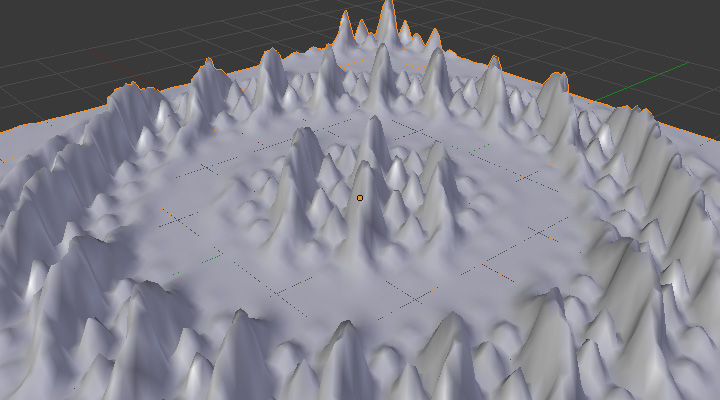# Vertex Weight Mix Modifier¶

This modifier mixes a second vertex group (or a simple value) into the affected vertex group, using different operations.

Important

This modifier does implicit clamping of weight values in the standard (0.0 to 1.0) range. All values below 0.0 will be set to 0.0, and all values above 1.0 will be set to 1.0.

Note

You can view the modified weights in Weight Paint Mode. This also implies that you will have to disable the Vertex Weight Mix modifier if you want to see the original weights of the vertex group you are editing.

## Options¶

Vertex Group A/B
A

The vertex group to affect.

B

The second vertex group to mix into the affected one. Leave it empty if you only want to mix in a simple value.

Invert Weights A/B

Invert the influence of the vertex group.

Default Weight A/B
A

The default weight to assign to all vertices not in the given vertex group.

B

The default weight to assign to all vertices not in the given second vertex group.

Vertex Set

Choose which vertices will be affected.

All

Affects all vertices, disregarding the vertex groups content.

Vertex Group A

Affects only vertices belonging to the affected vertex group.

Vertex Group B

Affects only vertices belonging to the second vertex group.

Vertex Group A or B

Affects only vertices belonging to at least one of the vertex groups.

Vertex Group A and B

Affects only vertices belonging to both vertex groups.

Important

When using All vertices, Vertices from group B or Vertices from one group, vertices might be added to the affected vertex group.

Mix Mode

How the vertex group weights are affected by the other vertex group’s weights.

Replace

Replaces affected weights with the second group’s weights.

Adds the values of Group B to Group A.

Subtract

Subtracts the values of Group B from Group A.

Multiply

Multiplies the values of Group B with Group A.

Divide

Divides the values of Group A by Group B.

Difference

Subtracts the smaller of the two values from the larger.

Average

Adds the values together, then divides by 2.

Normalize Weights

Scale the weights in the vertex group to keep the relative weight but the lowest and highest values follow the full 0 - 1 range.

### Influence¶

Those settings are the same for the three Vertex Weight modifiers, see the Vertex Weight Edit modifier page.

## Example¶

Here is and example of using a texture and the mapping curve to generate weights used by the Wave modifier.A customized mapping curve.¶Custom Mapping disabled.¶

The blend-file, TEST_4 scene.